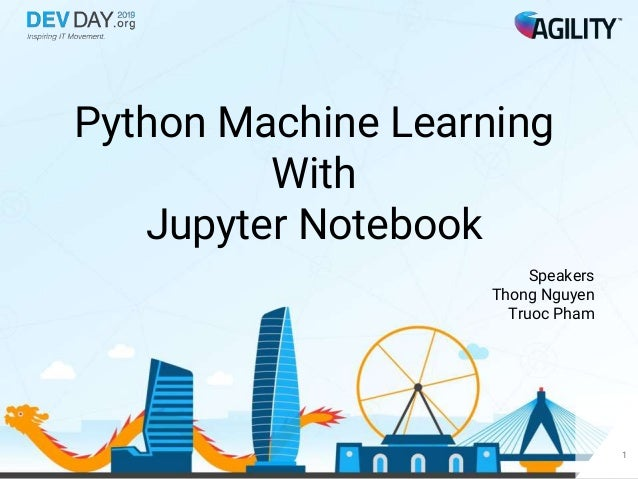Successfully reported this slideshow.Upcoming SlideShare
×

# [DevDay2019] Python Machine Learning with Jupyter Notebook - By Nguyen Huu Thong, Pham Khac Truoc, Developer at Agility IO

191 views

Published on

Their topic talks about what is Machine Learning and demo how to up and run Machine Learning on Jupyter Notebook.

Published in: Technology
• Full Name
Comment goes here.

Are you sure you want to Yes NoAre you sure you want to  Yes  No

### [DevDay2019] Python Machine Learning with Jupyter Notebook - By Nguyen Huu Thong, Pham Khac Truoc, Developer at Agility IO

1. 1. Python Machine Learning With Jupyter Notebook Speakers Thong Nguyen Truoc Pham 1
2. 2. Target & Audiences 2
3. 3. Agenda What is Machine Learning? How to get started? Our suggestion Simple Machine Learning project Q&A 3 1 2 3 4 5
4. 4. What is Machine Learning? 4 1
5. 5. 5 1
7. 7. 7 1
8. 8. Machine Learning is using data to answer questions 8 1
9. 9. How to get started? 9 2
10. 10. 10 Languages 2
11. 11. 11 2
12. 12. Our suggestion 12 3
13. 13. Python 13 # Python 3: Fibonacci series up to n >>> def fib(n): >>> a, b = 0, 1 >>> while a < n: >>> print(a, end=' ') >>> a, b = b, a+b >>> print() >>> fib(1000) 0 1 1 2 3 5 8 13 21 34 55 89 144 233 377 610 987 3
14. 14. 18K users 14 83% using Python https://www.kaggle.com/kaggle/kaggle-survey-2018/discussion/74297 3
15. 15. Libs for ML 15 3
16. 16. Jupyter Notebook 16 3
17. 17. 17https://www.kaggle.com/kaggle/kaggle-survey-2018/discussion/74297 3
18. 18. 18 3
19. 19. 19 3
20. 20. 20 3
21. 21. Simple Machine Learning project 21 4
22. 22. 22 4
23. 23. Demo https://devdayml2019.herokuapp.com/ 23 4
24. 24. 24 Data → Processing data → Training → Model → Serve Predictions 4
25. 25. 25 Preparing Data → Processing Data → Training → Model → Serve Predictions 4
26. 26. 26 Preparing Data → Processing Data → Training → Model → Serve Predictions Data for demo https://www.kaggle.com/c/house-prices-advanced-regression-techniques/data 4
27. 27. Preparing Data → Processing Data → Training → Model → Serve Predictions 27 - 1460 rows, 81 columns - Data types include: int64 float64 object 4
28. 28. Preparing Data → Processing Data → Training → Model → Serve Predictions 28 - Fill NaN - Outlier - Data Transformation 4
29. 29. Preparing Data → Processing Data → Training → Model → Serve Predictions 29 4
30. 30. 30 train = train.replace([-np.inf, np.inf], 0.0) train = train.fillna(0.0) test = test.replace([-np.inf, np.inf], 0.0) test = test.fillna(0.0) Preparing Data → Processing Data → Training → Model → Serve Predictions Sample code for filling NaN by Zero 4
31. 31. Preparing Data → Processing Data → Training → Model → Serve Predictions 31 4
32. 32. 32 train.drop( train[(train['GrLivArea'] > 4000)].index, inplace=True ) Preparing Data → Processing Data → Training → Model → Serve Predictions Sample code for removing outliers 4
33. 33. Preparing Data → Processing Data → Training → Model → Serve Predictions 33 4
34. 34. 34 def label_encoding(df): cat_features = df.select_dtypes(include=['object']).columns lbl = LabelEncoder() for col in cat_features: df[col] = lbl.fit_transform(list(df[col].values.astype('str'))) return df train = label_encoding(train) Preparing Data → Processing Data → Training → Model → Serve Predictions Sample code for label encoding 4
35. 35. Preparing Data → Processing Data → Training → Model → Serve Predictions 35 Linear regression 4
36. 36. 36 ignore_cols = ['Id', 'SalePrice'] train_features = [col for col in train.select_dtypes(include=['float64', 'int64']).columns if col not in ignore_cols] X = train[train_features].copy() y = np.log1p(train['SalePrice']) clf = LinearRegression() clf.fit(X, y) Using simple Linear Regression model 4 Preparing Data → Processing Data → Training → Model → Serve Predictions
37. 37. Preparing Data → Processing Data → Training → Model → Serve Predictions 37 Evaluation Model 4
38. 38. 38 def rmse_score(y_obs, y_hat): rmse = np.sqrt(mean_squared_error(y_obs, y_hat)) return rmse X_trn, X_val, y_trn, y_val = train_test_split( X, y, test_size=0.2, random_state=42) clf.fit(X_trn, y_trn) y_hat = clf.predict(X_val) rmse = rmse_score(y_val, y_hat) print('RMSE score: {:.6f}'.format(rmse)) Preparing Data → Processing Data → Training → Model → Serve Predictions Sample code for Evaluation Model 4
39. 39. Prepare Data → Processing Data → Training → Model → Serve Predictions 39 What is my house price? New Info ML Web Service Predicted Price 4
40. 40. Let’s getting started your wonderful Machine Learning project with Python & Jupyter Notebook 40 4
41. 41. References 41 http://bit.ly/agilityio-ml-2019 4
42. 42. Thank You 42 Speakers Thong Nguyen - nguyenthonght@gmail.com Truoc Pham - khactruoc09dce@gmail.com
43. 43. 43 5